Engineering ToolBox - Resources, Tools and Basic Information for Engineering and Design of Technical Applications!

# Pitot Tubes

## Pitot tubes can be used to measure fluid flow velocities by measuring the difference between static and dynamic pressure in the flow.

A pitot tube can be used to measure fluid flow velocity by converting the kinetic energy in a fluid flow to potential energy.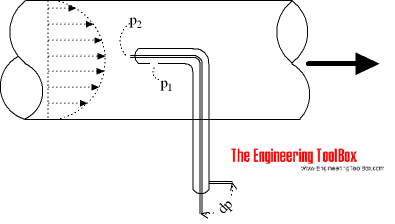The principle is based on the Bernoulli Equation where each term of the equation can be interpreted as pressure

p + 1/2 ρ v2 + ρ g h

=  p + 1/2 ρ v2 + γ h

= constant along a streamline                          (1)

where

p = static pressure (relative to the moving fluid) (Pa)

ρ = density of fluid (kg/m3)

v = flow velocity (m/s)

γρ g = specific weight (N/m3)

g = acceleration of gravity (m/s2)

h = elevation height (m)

Each term of the equation has the dimension force per unit area N/m2 (Pa) - or in imperial units lb/ft2 (psi).

### Static Pressure

The first term - p - is the static pressure. It is static relative to the moving fluid and can be measured through a flat opening in parallel to the flow.

### Dynamic Pressure

The second term - 1/2 ρ v2 - is called the dynamic pressure.

### Hydrostatic Pressure

The third term - γ h - is called the hydrostatic pressure. It represent the pressure due to change in elevation.

### Stagnation Pressure

The Bernoulli Equation states that the energy along a streamline is constant - and can be modified to

p1 + 1/2 ρ v12 + γ h1

= p2 + 1/2 ρ v22 + γ h2

= constant along the streamline                           (2)

where

suffix1 is a point in the free flow upstream

suffix2 is the stagnation point where the velocity in the flow is zero

### Flow Velocity

In a measuring point we regard the hydrostatic pressure as a constant where h1 = h2 - and this part can be eliminated. Since v2 is zero, (2) can be modified to:

p1 + 1/2 ρ v12 = p2                          (3)

or

v1 = [2 (p2 - p1) / ρ] 1/2

= [2 Δp / ρ] 1/2                (4)

where

Δp = p2 - p1  (differential pressure)

With (4) it's possible to calculate the flow velocity in point 1 - the free flow upstream - if we know the differential pressure difference Δp = p2 - p1 and the density of the fluid.

It is common to use head instead of pressure. (4) can be modified by dividing with specific weight γ to

v1 = c [2 g Δh]1/2                        (5)

where

c = coefficient - depending on reference liquid and units used or calculated

g = acceleration of gravity

Δh = h2 - h1 = height difference (fluid column)

Note! - in the basic equation the head unit is with reference to the density of the flowing fluid. For other units and reference liquids - like mm Water Column - check Velocity Pressure Head

### The Pitot Tube

The pitot tube is a simple and convenient instrument to measure the difference between static, total and dynamic pressure (or head).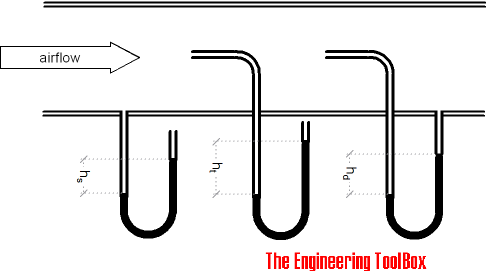The head - Δh - (or pressure difference - Δp) can be measured and calculated with the help of u-tube manometers, electronic pressure transmitters or similar instrumentation.

### Air Flow - Velocity and Dynamic Head Chart

The charts below are based on air density 1.205 kg/m3 and water density 1000 kg/m3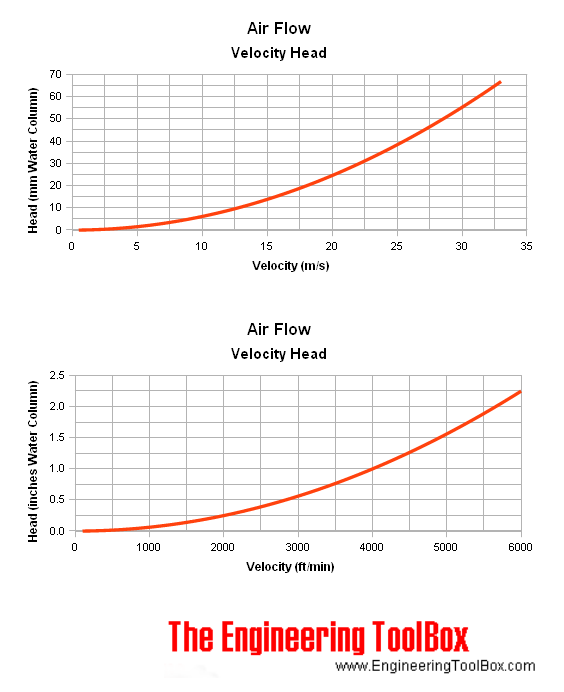Note that as indicated in the diagram above - pitot tubes are not suited for low velocity flow. Due to low dynamic pressure (head) the readings will be inaccurate.

### Water Flow - Velocity and Dynamic Head Chart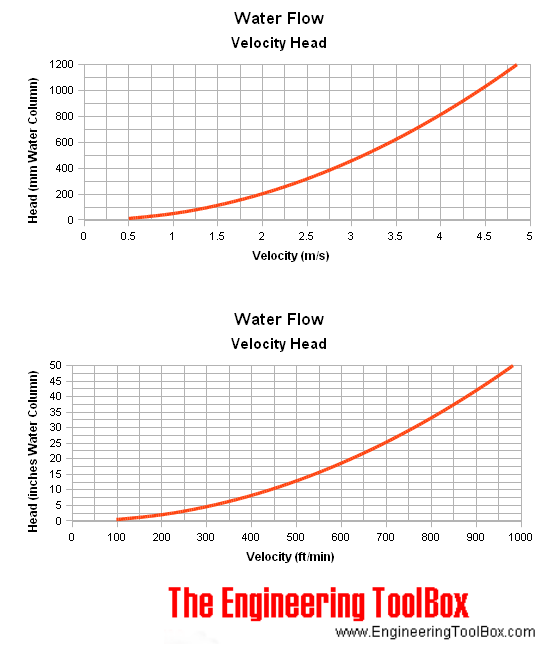### Flow Metering - Point Velocity Area Method

The point velocities in a duct, channel or pipe can be measured by traversing the cross-sectional area of the conduit. The point velocities can be used to calculate the average velocity that can be used to estimate the flow.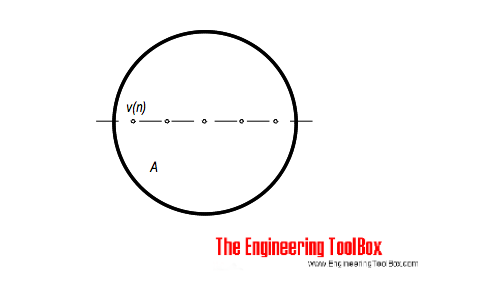The average velocity can be calculated as

va = Σ vn / n

= (2 g Σ hn / n )1/2              (6)

where

va = average velocity (m/s, ft/s)

vn = point velocity (m/s, ft/s)

hn = point height difference pitot meter  (m, ft)

n = number of point velocities

The volume flow in the conduit can be calculated as

q = va A                     (7)

where

q = flow (m3/s, ft3/s)

A = cross-sectional area (m2, ft2)

For round ducts larger than 10 inches the average velocity can be calculated as the arithmetic middle of a 10 point traverse from inward side wall with distance

• 2%, 8%, 15%, 23%, 34%, 66%, 77%, 85%, 92% and 98%

For smaller ducts the the average velocity can be estimated to 81% of centerline velocity.

## Related Topics

• Flow Measurement - Flow metering principles - Orifice, Venturi, Flow Nozzles, Pitot Tubes, Target, Variable Area, Positive Displacement, Turbine, Vortex, Electromagnetic, Ultrasonic Doppler, Ultrasonic Time-of-travel, Mass Coriolis, Mass Thermal, Weir V-notch, Flume Parshall and Sluice Gate flow meters and more.

## Engineering ToolBox - SketchUp Extension - Online 3D modeling!

Add standard and customized parametric components - like flange beams, lumbers, piping, stairs and more - to your Sketchup model with the Engineering ToolBox - SketchUp Extension - enabled for use with the amazing, fun and free SketchUp Make and SketchUp Pro .Add the Engineering ToolBox extension to your SketchUp from the SketchUp Pro Sketchup Extension Warehouse!

Translate

## Privacy

We don't collect information from our users. Only emails and answers are saved in our archive. Cookies are only used in the browser to improve user experience.

Some of our calculators and applications let you save application data to your local computer. These applications will - due to browser restrictions - send data between your browser and our server. We don't save this data.

## Citation

• Engineering ToolBox, (2003). Pitot Tubes. [online] Available at: https://www.engineeringtoolbox.com/pitot-tubes-d_612.html [Accessed Day Mo. Year].

Modify access date.

. .

#### Scientific Online Calculator3 10